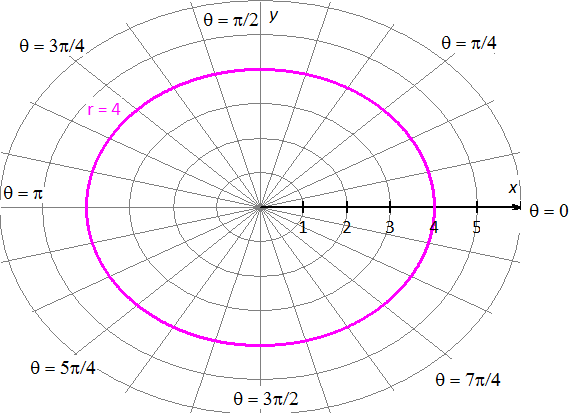PRE-ALGEBRA
ALGEBRA 1
GEOMETRY
ALGEBRA 2
TRIGONOMETRY
PRE-CALCULUS
CALCULUSPre-Calculus, 9th editionPAGE: 579 SET: Exercises PROBLEM: 13
Please look in your text book for this problem StatementThe polar equation is.

Convert the polar equation to a rectangular equation.Squaring on each side.Substitute..

The equation in rectangular form is.

The equationis in the form of standard circle equationwith centerand.Graph the equation.

Graph:.

The equationis circle with centerand radius.

Graph of the equation:TESTIMONIALS
 "I want to tell you that our students did well on the math exam and showed a marked improvement that, in my estimation, reflected the professional development the faculty received from you. THANK YOU!!!" June Barnett "Your site is amazing! It helped me get through Algebra." Charles "My daughter uses it to supplement her Algebra 1 school work. She finds it very helpful." Dan Pease
 Tweets by @mathskeydotcom

QUESTIONS? LET US HELP.
mathskey.com is not affiliated with any Publisher, Book cover, Title, Author names appear for reference only